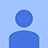# Đề thi Olympic Tiếng Anh lớp 10 vòng 2 năm học 2013 - 2014

1 493

## Đề thi Olympic Tiếng Anh lớp 10 vòng 2

Đề thi Olympic Tiếng Anh lớp 10 vòng 2 năm học 2013 - 2014. Đề thi đươc ra theo hình thức trắc nghiệm với 10 câu hỏi, phần đáp án đã được chúng tôi cập nhật đầy đủ và chính xác. Mời các bạn tham khảo.

Đề thi Olympic Tiếng Anh lớp 10 vòng 1 năm học 2013 - 2014

Đề thi Olympic Tiếng Anh lớp 10 vòng 1 năm học 2012 - 2013

Câu 1. Twenty-six squares of paper lettered A to Z are placed in a can. One letter is randomly chosen from the can and turns out to be the letter G. If a second letter is drawn without putting the letter G back into the can, what is the probability that it will be the letter F?

A. ¹⁄25 B. ¹⁄26 C. ²⁄25 D. ²⁄26 E. ³⁄25Câu 2. Only one of the equations below could possibly be the equation for this graph. Which one is it?

A. y = 2x + 3 B. y = 2x - 3
C. y = -3x - 2 D. y = 3x + 2
E. y = -3x + 2

Câu 3. Which of these is equivalent to the expression: x2 – 2x + 1, when x = –3?

A. -14 B. -2 C. 4 D. 8 E. 16

Câu 4. Which of these graphs does NOT represent a function?E. None of themCâu 5. A target has a center circle with a three-inch radius and five outer rings. Each ring is one inch wide. What is the circumference of the largest circle?

A. 6π inches B. 11π inches C. 20π inches
D. 18π inches E. 16π inchesCâu 6. What is the length of segment RS shown in the graph above?

A. 2√26 B. 2√34 C. 11 D. 12 E. 13

Câu 7. The mixed number 3¹⁄5 inches equals

A. 3 ÷ ¹⁄5 B. 3 - ¹⁄5 C. 3 x ¹⁄5 D. 3 + ¹⁄5 E. None of them

Câu 8. Simplify: 3x2 * (3x)2

A. 9x2 B. 18x4 C. 27x2 D. 27x3 E. 27x4

.2.086.19

Câu 9. Which list orders the numbers from the largest to the smallest?

A. .086, .19, .2 B. .19, .2, .086 C. .19, .086, .2
D. .2, .086, .19 E. .2, .19, .086

Câu 10. Suppose Martha has a job sewing zippers on down parkas. She gets 20 percent faster each day she works for the first ten days. If Martha can do 60 parkas the first day, about how many will she probably be able to handle by the end of the sixth day?